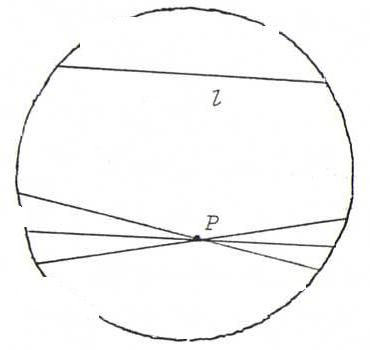### COURSE OF PROJECTIVE GEOMETRY

§ 7: answers

O27
a) Each plane through the normal of a plane α is perpendicular to α.
b) Two lines l and m through the origin in ℜ3are perpendicular if and only if m belongs to the plane α whereof l is a normal.
c) If l is a normal of α and m a normal of β, then l lies in β if and only if m lies in α.
d) If n runs through the plane γ, then the plane whereof n is a normal runs through the set of planes through the normal of γ.

O28 Beneath we have drawn three hyperbolic lines through P (in the circle model of the hyperbolic plane) that don't intersect l.O29 First the definitions of sinh and cosh: sinh(x) = (exp(x)-exp(-x))/2, cosh(x) = (exp(x)+exp(-x))/2.
The points (cosh(t),sinh(t)) run through one half of the hyperbola x2-y2=1, like (cos(t),sin(t)) run through the ellipse (circle) x2+y2=1.
Furthermore, d/dx sinh(x) = cosh(x) and d/dx cosh(x) = sinh(x), like d/dx sin(x) = cos(x) and d/dx cos(x) = -sin(x).
Now let f(x)=cosh(x) (x not negative). Because f ' isn't negative, and f(0)=1, f is at least 1 and a bijection from [0,∞) onto [1,∞). The inverse mapping is called arccosh.
Now we have to show: |p3q3-p1q1-p2q2| ≥ √(p32-p12-p22) √(q32-q12-q22) and ... = ... ⇔ p = q.
Define a symmetrical bilinear form by p.q := p3q3-p1q1-p2q2.
p+q).(λp+q) is positive if λp+q is lying inside k, 0 if λp+q is lying on k, and negative if λp+q is lying outside k. So if we choose two distinct fixed hyperbolic points p and q, then λ2p.p+2λp.q+q.q has two distinct zeros, and then the discriminant is positive.

O30 Let P = λ(0,0,1) and Q = λ(a,0,1). Then d(P,Q) = arccosh(1/√(1-a2)) → ∞ als a ↑ 1.

O31 Consider P2 with in it the circle x2+y2=z2. Let X=(xo,yo,zo).
The polar line of P is the line with equation xxo+yyo=zzo.
We have: P is on the polar line of Q ⇔ xPxQ+yPyQ=zPzQQ is on the polar line of P.
Furthermore: if X lies on the circle, then the polar line of X is tangent to the circle in X.
So, in the picture, P lies on the polar lines of both intersection points of l and the circle, so l is the polar line of P.
Hence, the equation of l is xxP+yyP=zzP.
Now let n be a hyperbolic line "through P". Then the equation of n has the form ax+by=cz where axP+byP=czP.
Since l has projective coordinates (xP,yP,-zP), and n has projective coordinates (a,b,-c), whilst czP-axP-byP=0, we find lm.